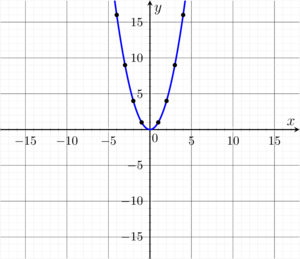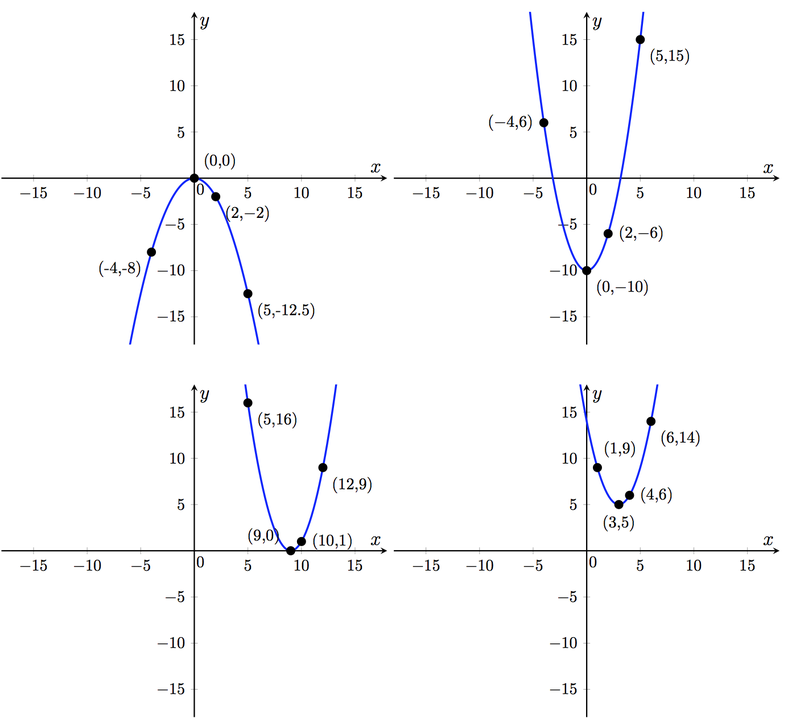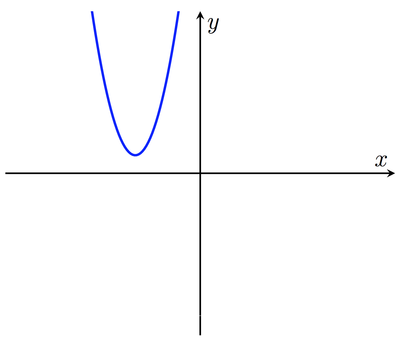# Identifying Quadratic Functions (Standard Form)

Alignments to Content Standards: F-BF.B.3 A-SSE.B.3

The figure shows a graph of the function $f(x)=x^2$.a)  For each of the graphs of quadratic functions below, find values of $a$, $b$, and $c$ so that the function $f(x)=ax^2+bx+c$ has that graph. (For example, the graph in the first part corresponds to $a=1$, $b=0$, and $c=0$).b)  For each of the following three descriptions of graphs of quadratic functions, sketch a graph by hand, and then find a function in the form $f(x)=ax^2+bx+c$ whose graph fits the description.

• The graph is concave down and has its vertex at $(0,7)$.
• The graph is concave up and has its vertex at $(-7,0)$.
• The graph has its vertex at $(4,-9)$ and passes through the point $(6,-1)$.

c) If the graph of the function $f(x)=ax^2+bx+c$ is as below, determine the signs of $a$, $b$, and $c$.## IM Commentary

This task has students explore the relationship between the three parameters $a$, $b$, and $c$ in the equation $f(x)=ax^2+bx+c$ and the resulting graph. There are many possible approaches to solving each part of this problem, especially the first part. We outline some of them here (which overlap heavily in places), applied to the top left graph, and then only give the final answers in the solution below:

1. Transformational/graphical approach: The graph is a scaled reflection of the graph of $y=x^2$ over the $x$ axis, and so takes the form $y=ax^2$ for some negative value of $a$. Taking a single point on the graph, say $(5,-12.5)$, means we must have $-12.5=a(5^2)$, giving $a=-\frac{1}{2}$.
2. Graphical inspection/vertex formula: Since the graph goes through the origin, we have $c=f(0)=0$. Further, since the vertex of the graph occurs with an $x$-coordinate of $-\frac{b}{2a}$, and in this case occurs at $x=0$, we must have $b=0$. So we have $f(x)=ax^2$ for some value of $a$ and proceed as in the previous part.
3. Systems of equations approach: For the algebraically fluent, taking three input-output pairs gives three equations which one can solve for $a$, $b$, and $c$. For example, taking $f(0)=0$, $f(-2)=-2$, and $f(2)=-2$
\begin{align}
0&=a(0)^2+b(0)+c\\
-2&=a(-2)^2+b(-2)+c\\
-2&=a(2)^2+b(2)+c
\end{align}
The first gives $c=0$ immediately, and it is easy to solve the last two to get $b=0$ and $a=-\frac{1}{2}$.
4. Experimental approach: Students might open a new worksheet in, for example, desmos.com, and drag sliders for the coefficients to make the graphs fit the points exactly. We caution that entering points might be somewhat time-consuming, and so teachers should use this approach with discretion -- in particular, it may be worth relying on the fact that it only requires three points to determine a parabola!

Notice that fluency converting between different forms of an expression is useful in solving the task. For example, the solution to the third graph in part (a), $a=1$, $b=-18$, and $c=81$, is difficult to find by trial and error using a graphing utility, but is easy if you recognize the graph as that of $f(x)=(x-9)^2$, obtained by translating the graph of $f(x)=x^2$ nine units to the right. This is made even more explicit in the last part, where it is crucial to see how the coordinates of the vertex and the coefficients of the quadratic in standard form relate to one another.

Finally, we note that the task uses the phrases "concave up" and "concave down" rather than "upward-facing" and "downward-facing" parabolas.  This terminology should be introduced if new to the students.

## Solution

1)  The four graphs are (from top left to bottom right)

• $f(x)=-\frac{1}{2}x^2$, so $a=-\frac{1}{2}$, $b=0$, and $c=0$.
• $f(x)=x^2-10$, so $a=1$, $b=0$, and $c=-10$.
• $f(x)=(x-9)^2=x^2-18x+81$, so $a=1$, $b=-18$, and $c=81$.
• $f(x)=(x-3)^2+5=x^2-6x+14$, so $a=1$, $b=-6$, and $c=14$.

2)

• One example is $f(x)=-x^2+7$.
• One example is $f(x)=(x+7)^2 = x^2+14x+49$.
• Any such example takes the form $f(x)=a(x-4)^2-9$ for some constant $a$. To ensure that $f(6)=-1$, we need to ensure that $a(6-4)^2-9=-1$, which forces $a=2$.

3) Of course, without any scales on the axes, it will be impossible to figure out exact values for these coefficients. But as it turns out, we'll be able to reason whether each one of the coefficients is positive or negative. Writing $f(x)=ax^2+bx+c$, we first see that since the given parabola is upward-facing, we must have $a>0$. Next, by its location in the second quadrant we can also see that the coordinates of the vertex $(h,k)$ satisfy $h<0$ and $k>0$. How does this help with $a$, $b$, and $c$? Well, if we write $f(x)$ in the form $$f(x)=a(x-h)^2+k=ax^2-2ahx+(h^2+k),$$ we see that the two sets of coefficients are related by $b=-2ah$ and $c=h^2+k$. Now we see that the linear coefficient $b=-2ah$ must be positive (since $a$ is positive and $h$ is negative). Similarly, the constant coefficient $c=h^2+k$ must be positive (since both $h^2$ and $k$ are positive).